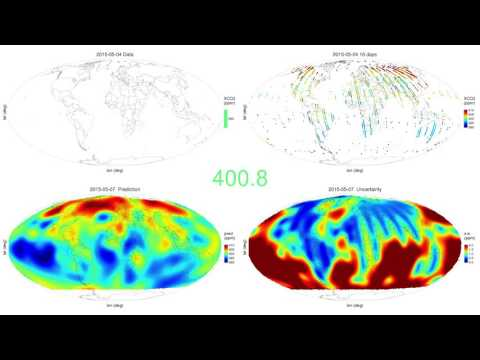The package FRK is available on CRAN! To install, please type

install.packages("FRK")

To install the most recent development version, first please install INLA from https://www.r-inla.org/download, then please load devtools and type

install_github("andrewzm/FRK", dependencies = TRUE, build_vignettes = TRUE)

A paper introducing the package is available here. A paper detailing the approach in a non-Gaussian setting is available here (a six-page summary of this paper is available here).

The vignette “FRK_intro” summarises the package, gives details on the EM algorithm that may be employed in a Gaussian setting, and provides several examples. Another vignette, “FRK_non-Gaussian”, summarises inference in a non-Gaussian setting (where a Laplace approximation is used), and contains examples using non-Gaussian data and the newly available plotting methods. To access the vignettes, please click on the following links:

Introduction to FRK

Tutorial on modelling spatial and spatio-temporal non-Gaussian data with FRK

A pkgdown page is also available here.

## Supporting and citing

If you use FRK in your work, research, or other activities, please cite it using the information provided by citation("FRK").

## Description

Package: FRK

Type: Package

Title: Fixed Rank Kriging

Version: 2.1.5

Date: 2023-01-30

Author: Andrew Zammit-Mangion, Matthew Sainsbury-Dale

Maintainer: Andrew Zammit-Mangion

Description: A tool for spatial/spatio-temporal modelling and prediction with large datasets. The approach models the field, and hence the covariance function, using a set of basis functions. This fixed-rank basis-function representation facilitates the modelling of big data, and the method naturally allows for non-stationary, anisotropic covariance functions. Discretisation of the spatial domain into so-called basic areal units (BAUs) facilitates the use of observations with varying support (i.e., both point-referenced and areal supports, potentially simultaneously), and prediction over arbitrary user-specified regions. FRK also supports inference over various manifolds, including the 2D plane and 3D sphere, and it provides helper functions to model, fit, predict, and plot with relative ease. Version 2.0.0 and above also supports the modelling of non-Gaussian data (e.g., Poisson, binomial, negative-binomial, gamma, and inverse-Gaussian) by employing a generalised linear mixed model (GLMM) framework. Zammit-Mangion and Cressie doi:10.18637/jss.v098.i04 describe FRK in a Gaussian setting, and detail its use of basis functions and BAUs, while Sainsbury-Dale et al. <arXiv:2110.02507> describe FRK in a non-Gaussian setting; two vignettes are available that summarise these papers and provide additional examples.

• Zammit-Mangion, A. & Cressie N. (2021). “FRK: an R package for spatial and spatio-temporal prediction with large datasets.” Journal of Statistical Software, 98, 1-48.
• Sainsbury-Dale, M., Zammit-Mangion, A. & Cressie, N. (2023). “Modelling Big, Heterogeneous, Non-Gaussian Spatial and Spatio-Temporal Data using FRK” Journal of Statistical Software, accepted for publication, https://arxiv.org/abs/2110.02507.

## Quick start

### Gaussian data

library("FRK")
library("sp")
library("ggplot2")
library("ggpubr")

## Setup
m <- 1000                                                  # Sample size
RNGversion("3.6.0"); set.seed(1)                           # Fix seed
zdf <- data.frame(x = runif(m), y= runif(m))               # Generate random locs
ggarrange(plotlist$z, plotlist$p_mu, plotlist$interval90_mu, nrow = 1, legend = "top")(Left) Poisson data. (Centre) Prediction of the mean response. (Right) Prediction interval width of the mean response. ### Spatio-temporal data We now analyse spatio-temporal data, using the NOAA dataset. ## Setup library("spacetime") data("NOAA_df_1990") Tmax <- subset(NOAA_df_1990, month %in% 7 & year == 1993) Tmax <- within(Tmax, {time = as.Date(paste(year,month,day,sep="-"))}) STObj <- stConstruct(x = Tmax, space = c("lon","lat"), time = "time", interval = TRUE) ## BAUs: spatial BAUs are 1x1 pixels, temporal BAUs are 1 day intervals BAUs <- auto_BAUs(manifold = STplane(), cellsize = c(1, 1, 1), data=STObj, tunit = "days") BAUs$fs <- 1 # scalar fine-scale variance matrix, implicit in previous examples

## Basis functions
G <- auto_basis(manifold = STplane(), data = STObj, nres = 2, tunit = "days")

## Run FRK
STObj$std <- 2 # fix the measurement error variance S <- FRK(f = z ~ 1 + lat, data = list(STObj), basis = G, BAUs = BAUs, est_error = FALSE, method = "TMB") pred <- predict(S, percentiles = NULL) ## Plotting: include only some times via the argument subset_time plotlist <- plot(S, pred$newdata, subset_time = c(1, 7, 13, 19, 25, 31))
ggarrange(plotlist = plotlist, nrow = 1, legend = "top")(Left) Prediction of spatio-temporal process. (Right) Prediction interval width.

## Demonstrations

The package FRK is currently being used to generate spatio-temporal animations of fields observed by satellite data. Here we show a daily prediction of CO2 using data from the NASA OCO-2 between September 2014 and June 2016.## Acknowledgements

Thanks to Michael Bertolacci for designing the FRK hex logo!